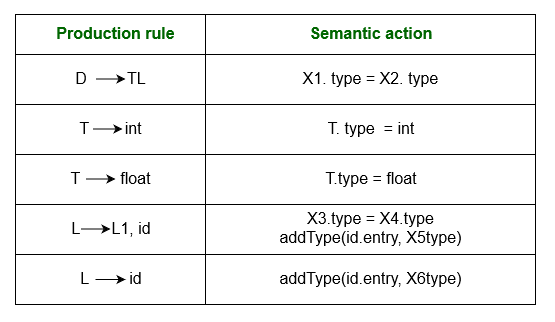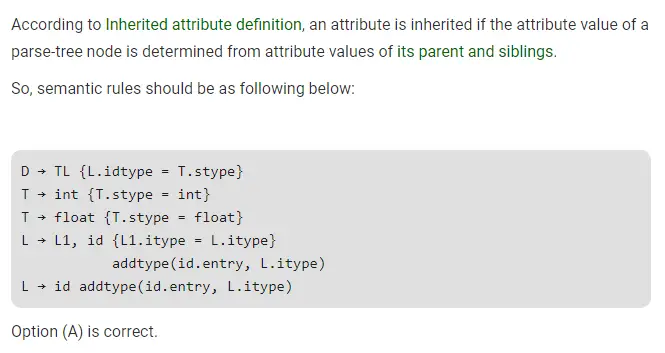# Gate CS-2019 Question Paper With Solutions

Q. 45 Consider the following grammar and the semantic actions to support the inherited type declaration attributes. Let X1, X2, X3, X4, X5 and X6 be the placeholders for the non-terminals D, T, L or L1 in the following table:Which one of the following are the appropriate choices for X1, X2, X3 and X4?

(A) X1 = L, X2 = T, X3 = L1, X4 = L

(B) X1 = L, X2 = L, X3 = L1, X4 = T

(C) X1 = T, X2 = L, X3 = L1, X4 = T

(D) X1 = T, X2 = L, X3 = T, X4 = L1